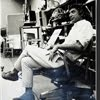## Eugene Ray SDSU 1970'sEugene Ray 1970's. photo credit: Tom Davis

## Tuesday, January 22, 2013

### VITAL BRITISH & JAPANESE links> reyner banham/minoru takeyama speakers were important @ sdsu ^^^^^^^^^^^^^^^^^^^^^ YOSHIKI MATSUHISA, MFA, SDSU alumnus now professor in japan ! ^^^^^^^^^^^^^^^^^^^^^^ ENVIRONMENTAL THEORY CLASS @sdsu was projenitor of this blog ^^^^^^^^^^^^^^^^^^^^^^ DESIGN RESEARCH gave us FAME for cutting edge ARCHITECTURE>were important to our<
>sdsu design program<
^^^^^^^^^^^

+REYNER BANHAM+
^^^^^^^^^^^^^^^^^
+MINORU TAKEYAMA+
^^^^^^^^^^^^^^^^^
+WERE PREMIER SPEAKERS+
>world class<
+CUTTING EDGE THOUGHT+
^^^^^^^^^^^^^^

>prof. yoshiki matsuhisa mfa/ed sdsu<
>now professor of landscape design<
+DISTINGUISHED+
>at a university in osaka japan<
^^^^^^^^^^^^^^^
+DESIGN RESEARCH+
>was important to our sdsu/ed<
>cutting edge architecture<
^^^^^^^^^^^^^

+TONIGHT'S PHOTOS+
>research as the basis for leading<
>edge position in environ design<
^^^^^^^^^^^^^^^^^^

#1)             +REYNER BANHAM+
>prof. of arch, u.c. santa cruz<
^^^^^^^^^^^^^^
>architectural historian and<
+INTERNATIONAL+
>speaker of our years at<
>san diego state univ<
^^^^^^^^^^

#2)        +THEORY CLASS=SDSU+
>reyner gave a major evening public<
>lecture at sdsu and this seminar<
>in my environ theory class<
^^^^^^^^^^^^^^
+FIRST MACHINE AGE+
>and<
+LOS ANGELES ARCHITECTURE+
+OF THE FOUR ECOLOGIES+
^^^^^^^^^^^^^^
(>two of his great books<
^^^^^^^^
>reyner died not long after his visit<
>but mary, his widow came to<
+GOOD FRIENDS+
>both of my london lectures<
^^^^^^^^^^^^^^^

#3)          +MINORU TAKEYAMA+
>of cutting edge architecture<
^^^^^^^^^^^^^^^
>worked for him in tokyo made<
+SLIDE LECTURE+
>a major public presentation<
>and seminar in my class<
^^^^^^^^^^^^^
+MINORU ALSO EXHIBITED+
>sdsu/arch. show<
+IN "ECCENTRIC PLACES"+
^^^^^^^^^^^^

#4)            +NIKOLA TESLA+
>mistreated bypassed technical genius<
>of nikola tesla was typical research<
>in our environmental theory<
^^^^^^^^^^^^
+TESLA HAS FINALLY BEEN+
+RECOGNISED FOR HIS+
+GREAT GENIUS+
^^^^^^^
>the agents of thomas edison have<
>been revealed for unfair play<
>regarding some inventions<
>(international activity)<
^^^^^^^^^^^

#5)       +YOSHIKI MATSUHISA+

^^^^^^^^^^^^^^^
>our distinguished sdsu/e.d. mfa<
>alum now professor of design<
>at the university of osaka<
^^^^^^^^^^^^^^
+WAS THE FIRST TO SEND+
>thank yoshi<
+ME GREETINGS FOR+
+YEAR 2000+ !
^^^^^
>yoshi's sdsu/e.d. thesis project was<
>an excellent system of affordable<
+EXPANDABLE SYSTEM+
>mass production housing with<
>a kit of parts design concept<
^^^^^^^^^^^^^^^^

+THESE FOND MEMORIES+
>evening sky<
+ENLIGHTEN OUR LIVES+
^^^^^^^^^^

gene ray, lajolla, california

>eugene ray, mfa, architect<
>professor emeritus, sdsu<
^^^^^^^^^^^^^^